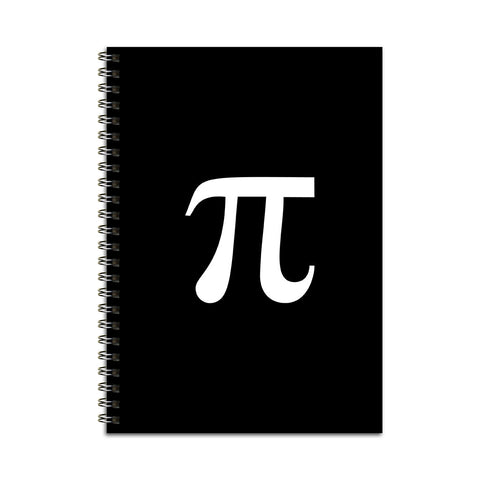Browse More:

Black & White Geek Pi

Pi Notebook

• \$18.00

Pi is a mathematical constant and a transcendental (and therefore irrational) real number, approximately equal to 3.14159, which is the ratio of a circle's circumference to its diameter in Euclidean geometry, and has many uses in mathematics, physics, and engineering. It is also known as Archimedes' constant (not to be confused with an Archimedes number) and as Ludolph's number.

Pi (to 50 decimal places) =

3.14159 26535 89793 23846 26433 83279 50288 41971 69399 37510

æ

You might also like ...